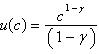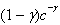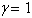/
/
/
SHORT ANSWER 1.A widely used utility function in the economics literature
Not my Question
Flag Content

# Question : SHORT ANSWER 1.A widely used utility function in the economics literature : 2081469

1.A widely used utility function in the economics literature is the constant rate of risk aversion utility function, of which log utility is a special case. It is given by.With this utility function, the marginal utility of consumption is. What is the Euler equation for this utility function? Show that if, the Euler equation is the same as if we used log utility.

## Solution 5 (1 Ratings )

Solved
Economics 3 Days Ago 34 Views